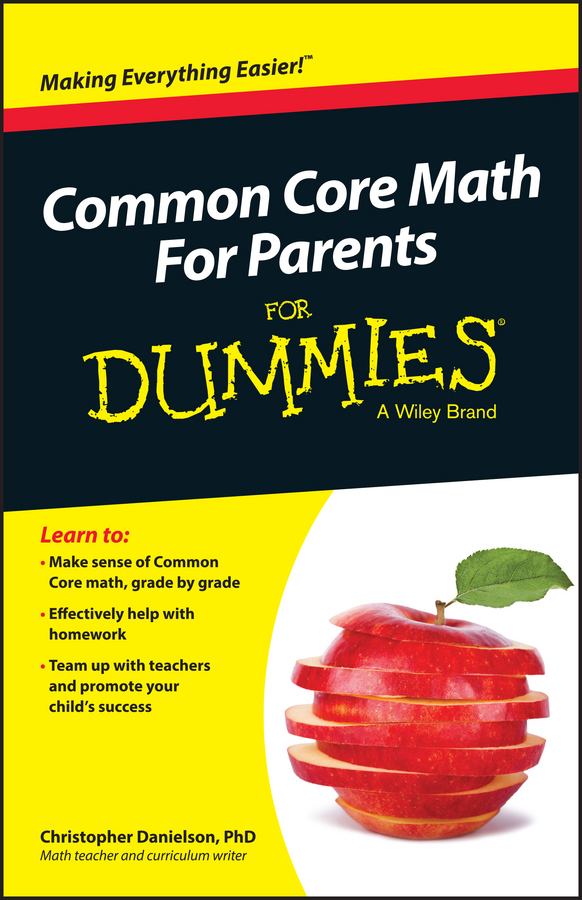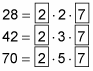##### Common Core Math For Parents For Dummies with Videos OnlineThe greatest common factor (GCF) of a set of numbers is the largest number that is a factor of all those numbers. For example, the GCF of the numbers 4 and 6 is 2 because 2 is the greatest number that’s a factor of both 4 and 6. Here you will learn two ways to find the GCF.

## Method 1: Use a list of factors to find the GCF

This method for finding the GCF is quicker when you’re dealing with smaller numbers. To find the GCF of a set of numbers, list all the factors of each number. The greatest factor appearing on every list is the GCF. For example, to find the GCF of 6 and 15, first list all the factors of each number.

Factors of 6: 1, 2, 3, 6

Factors of 15: 1, 3, 5, 15

Because 3 is the greatest factor that appears on both lists, 3 is the GCF of 6 and 15.

As another example, suppose you want to find the GCF of 9, 20, and 25. Start by listing the factors of each:

Factors of 9: 1, 3, 9

Factors of 20: 1, 2, 4, 5, 10, 20

Factors of 25: 1, 5, 25

In this case, the only factor that appears on all three lists is 1, so 1 is the GCF of 9, 20, and 25.

## Method 2: Use prime factorization to find the GCF

You can use prime factorization to find the GCF of a set of numbers. This often works better for large numbers, where generating lists of all factors can be time-consuming.

Here’s how to find the GCF of a set of numbers using prime factorization:

1. List the prime factors of each number.

2. Circle every common prime factor — that is, every prime factor that’s a factor of every number in the set.

3. Multiply all the circled numbers.

The result is the GCF.

For example, suppose you want to find the GCF of 28, 42, and 70. Step 1 says to list the prime factors of each number. Step 2 says to circle every prime factor that’s common to all three numbers (as shown in the following figure).As you can see, the numbers 2 and 7 are common factors of all three numbers. Multiply these circled numbers together:

2 · 7 = 14

Thus, the GCF of 28, 42, and 70 is 14.

Knowing how to find the GCF of a set of numbers is important when you begin reducing fractions to lowest terms.